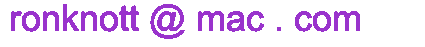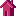## A General Fibonacci Number Calculator version 3.1 (September 2016)

This multi-precision Calculator will find terms of a General Fibonacci Sequence G which has two given starting values: G(0)=a and G(1)=b and then uses the Fibonacci Rule: "add the two previous values to get the next" with the sequence extending backwards too (for negative indices).
We write G(a,b,i) for the i-th term but a and b will be constant for a particular G series. The Fibonacci numbers F(i) are G(0,1,i) and the Lucas numbers L(i) are G(2,1,i).
The Calculator can produce numbers with thousands of decimal digits, thanks to the BigNumber Javascript functions package.
for example: Fib(9 007 199 254 740 991) has 1 88239 33175 09687 digits. Spaces in the input box numbers are ignored.
Click on the title links to go to an introductory section of a page on this site on that topic.
Fibonacci numbers F(i) = G(0,1,i)
0,1,1,2,3,5,8,13,21,...
Lucas numbers L(i) = G(2,1,i)
2,1,3,4,7,11,18,29,...
Generalised Fibonacci numbers G(a,b,i)
starting from any two given numbers, a and b, use the Fibonacci Rule: add the previous two numbers to get the next:
G(0) = a, G(1) = b, G(a,b,n) = G(a,b,n-1) + G(a,b,n-2) for all integers n.
the number of digits
Can compute the number of digits in huge G series numbers - see the previous section.
exact remainders mod n
finds the remainders on division by a given number n ("modulo n" or "mod n" for short). This is always exact for any index number i.
Benford's Law Plots
Benford's Law states that the initial digit of many mathematical series as well as natural data (such as populations of countries and sizes of lakes) have a precise rule that determines how popular is the first digit. 1 is the most popular, 2 is less popular through to the least popular initial digit: 9. Here you can plot initial digits or the first two digits for a range of values in any G series and examine the data for yourself, shown as a frequency bar chart of the first one or the first two digits of the Fibonacci, Lucas of General Fibonacci function in the given range of indices.
The output also shows the list of frequencies for first digits 1-9 or first two digits 10-99 which is ready for copying into a spreadsheet for further investigation.
Pisano periods and Entry points
The Mathematics of the Fibonacci Numbers page has a section on the periodic nature of the remainders when we divide the Fibonacci numbers by any number (the modulus). The Calculator on this page lets you examine this for any G series.
Also every number n is a factor of some Fibonacci number. But this is not true of all G series. The first G series number which has n as a factor is called the Entry Point of n in that series.
the Rabbit Sequence, Rab(i)
also called the Fibonacci Word and the Golden String
This sequence of bits is related to the Powers of Phi, the golden ratio which is Phi = (√5 + 1)/2 = 1.6180339... and phi = 1/Phi = Phi−1 = (√5 − 1)/2 = 0.6180339... and also to multiples of Phi.
(0)101101011011... indexed as follows:
 i Rab(i) 0 1 2 3 4 ... 0 1 0 1 1 0 1 0 1 1 0 1 1
The Amaze Me! button
The button finds an amazing formula that looks impossible but is true. For example
Approximate values are calculated immediately for any size of index, larger values being given in a scientific notation, for example:
1234 is 1.234×103.

For very large numbers, you can specify the number of digits per line and indicate if you want them broken up into 5-digit blocks with a space separator too.

C A L C U L A T O R for Fibonacci and General Fibonacci (G) Sequences
G(a,b,0)=a,  G(a,b,1)=b,  G(a,b,i) = G(a,b,i−1) + G(a,b,i−2) for any integer i
the General Fibonacci series  action buttons

G(a,b,0) = a =
G(a,b,1) = b =

for i =
up to

for
up to
 Rabbit Sequence (Fibonacci Word) and Phi  for i=  up to

R E S U L T S        For large numbers: number of digits per line=   with 5-digit blocks separated:Fibonacci Home Page